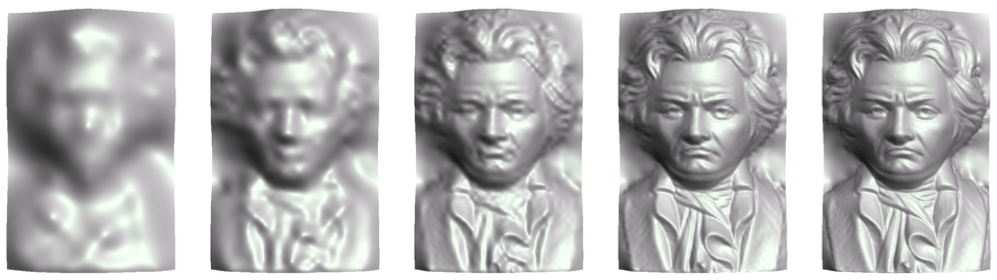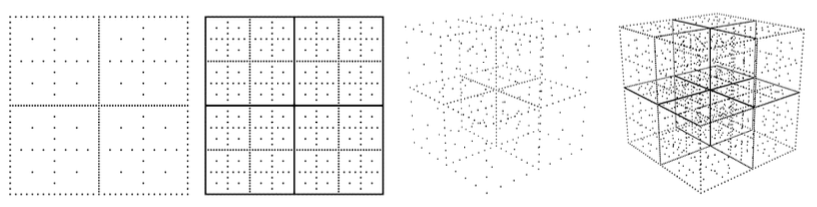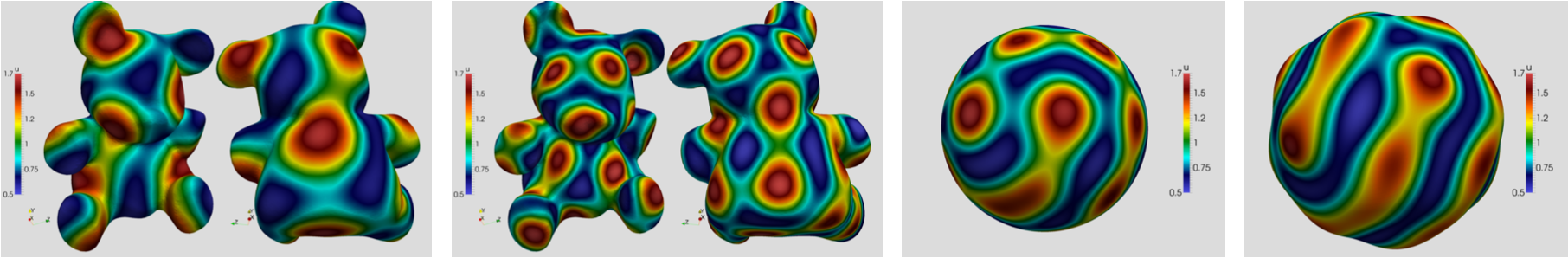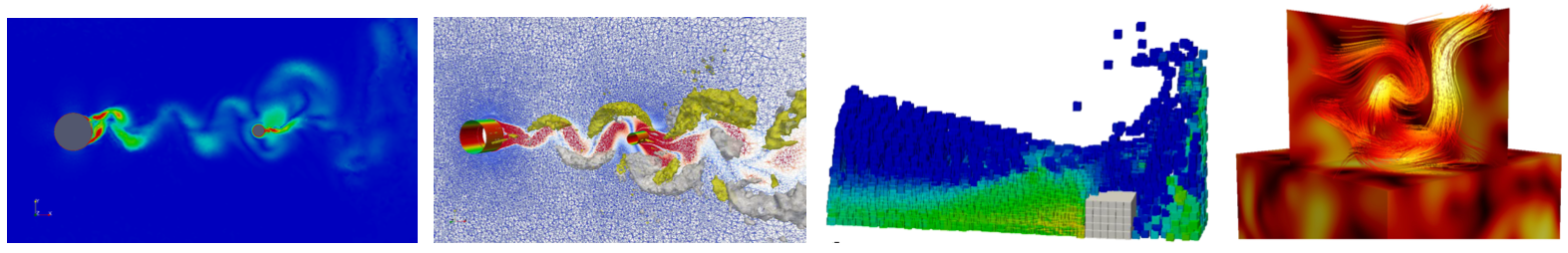Print page

# Research

The research at our chair is concerned with topics from applied mathematics, numerical analysis and scientific computing. In particular, we develop, analyse and validate current methods in multivariate and high-dimensional approximation theory. The focus is on meshfree, kernel-based methods such as radial basis functions, moving least-squares and particle methods. We are also interested in applications of these methods, for exampe for solving partial differential equations, within learning theory and data analysis.

Recent projects comprise:

1. Multivariate approximation theory with radial basis functions, moving-least squares and deep neural networks. We develop data driven, stable and efficient methods for computing approximations in low-dimensional spaces.  For example, multilevel methods with compactly supported radial basis functions are developed and analysed. In contrast to other multiscale methods as, for example, wavelets, such methods work with arbitrary data sets on arbitrary domains.2.
3. Kernel-based multilevel methods for high-dimensional approximation problems. Besides classical techniques as radial basis functions, we analyse and validate combinations of such techniques with so-called sparse grids. In contrast to classical mesh-based methods, such techniques allow us to weaken the curse of dimensionality, which makes these methods feasible for moderately high-dimensional problems.4.
5. Solving partial differential equations on surfaces and bounded domains. Here, we employ once again discretisation techniques based on radial basis functions, as they only rely on point-based information. They are particularly favourable if the underlying geometry is changing or if only incomplete data are given. We use and analyse methods based on Galerkin and collocation techniques.6.
7. Meshfree methods for numerically solving problems from fluid dynamics. We develop numerical solution methods for classical problems from fluid dynamics. We are particularly interested in problems with free boundaries. The methods employ kernel-based discretisations and are based on either an Eulerian or a Lagrangian point of view.  In particular, we study particle-based methods such as SPH (smoothed particle hydrodynamics). Moreover, we are interested in efficient methods for fluid-structure-interaction problems.Webmaster: Univ.Prof.Dr. Holger Wendland